使用java,生成不带指定数字的序号

【推荐阅读】滴滴出行的数据架构和信息流处理思路解析->>>一般的订单号是有规则的如：170081300002760 其中红色部分是使用seq生成的。

0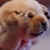引用来自“明月照大江”的答案

0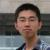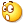0~9排开4的char[] 按9进制取对应位置元素生成String就可以了吧...
0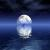0引用来自“明月照大江”的答案0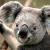0引用来自“明月照大江”的答案

python代码如下：

#!/usr/bin/python
# Filename:policyCode.py

def createCode(num):
str = ["0","1","2","3","5","6","7","8","9"]
result = []
str2 =""
isRunning = True
while isRunning:
n = num%9
#print str[n],
result.append(str[n])
num = num/9
if num <=0:
isRunning = False
else:
r = result[::-1]  #sort list by desc
#print r
for item in r:
str2+=item
return str2

for i in range(0,999):
f = file('list.txt','a') # append str to file
str = createCode(i)
f.write(str+"\n")
f.close()
print 'DONE.'

1 == 0

2 == 1

3 == 2

4 == 3

5 == 5

6 == 6

7 == 7

8 == 8

9 == 9

....

82 == 100

83 == 101